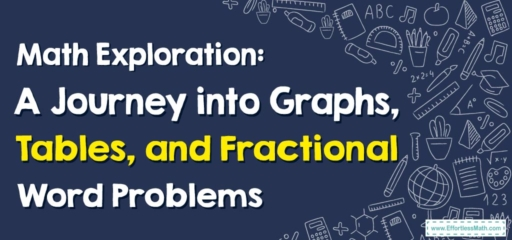# Math Exploration: A Journey into Graphs, Tables, and Fractional Word Problems

Greetings, math explorers!
Today, we're embarking on an exciting expedition into the land of Graphs and Tables. Our mission? To solve intriguing word problems involving fractions. So, strap on your boots, grab your compass, and let's begin our adventure!## 1. The Adventure Land: Graphs, Tables, and Fractions

Our destination is filled with graphs and tables that help us visualize and manage data. Our challenges along the way will be word problems involving fractions, those tricky, half-and-quarter-sized math creatures.

## 2. The Expedition: Solving Word Problems

Solving word problems requires careful reading, identifying the information given, and determining the process needed to find the solution. But with graphs, tables, and our explorer spirit, we’ll be conquering these problems in no time.

### Your Adventurer’s Guide: How to Use Graphs and Tables to Solve Fractional Word Problems

Here’s the path:

#### Step 1: Understanding the Problem

Read the problem carefully. What is it asking you to find? What information is provided?

#### Step 2: Organizing the Data

Use a table to organize the data. This helps you see the relationships between different parts of the problem.

#### Step 3: Graphing the Data

Sometimes, drawing a graph can provide a visual representation of the problem, making it easier to see the solution.

#### Step 4: Solve the Problem

Apply mathematical operations as required. In this case, you’ll likely be using operations involving fractions.

For example, if a word problem states: “Lily has $$\frac{3}{4}$$ of a cake and eats $$\frac{2}{8}$$ of it. How much cake does she have left?”

1. Understanding the Problem: Lily starts with $$\frac{3}{4}$$ of a cake and eats some. We need to find out how much she has left.
2. Organizing the Data: You could make a table with two columns: Cake at Start ($$\frac{3}{4}$$) and Cake Eaten ($$\frac{2}{8}$$).
3. Graphing the Data: You could draw a pie graph representing the whole cake, shade $$\frac{3}{4}$$ to show what Lily had initially, and then shade $$\frac{2}{8}$$ in a different color to show what she ate.
4. Solve the Problem: Subtract $$\frac{2}{8}$$ from $$\frac{3}{4}$$ to find out how much cake Lily has left. But remember to convert them to have the same denominator first! So, $$\frac{3}{4}\ – \frac{2}{8} = \frac{6}{8}\ – \frac{2}{8} = \frac{4}{8}$$, which simplifies to $$\frac{1}{2}$$.

And there we have it, brave explorers! We’ve successfully navigated the terrain of Graphs, Tables, and Fractional Word Problems. Remember, each math problem is a new adventure waiting to be discovered. So, keep exploring, and see you on our next math expedition!

### What people say about "Math Exploration: A Journey into Graphs, Tables, and Fractional Word Problems - Effortless Math: We Help Students Learn to LOVE Mathematics"?

No one replied yet.

X
30% OFF

Limited time only!

Save Over 30%

SAVE $5 It was$16.99 now it is \$11.99